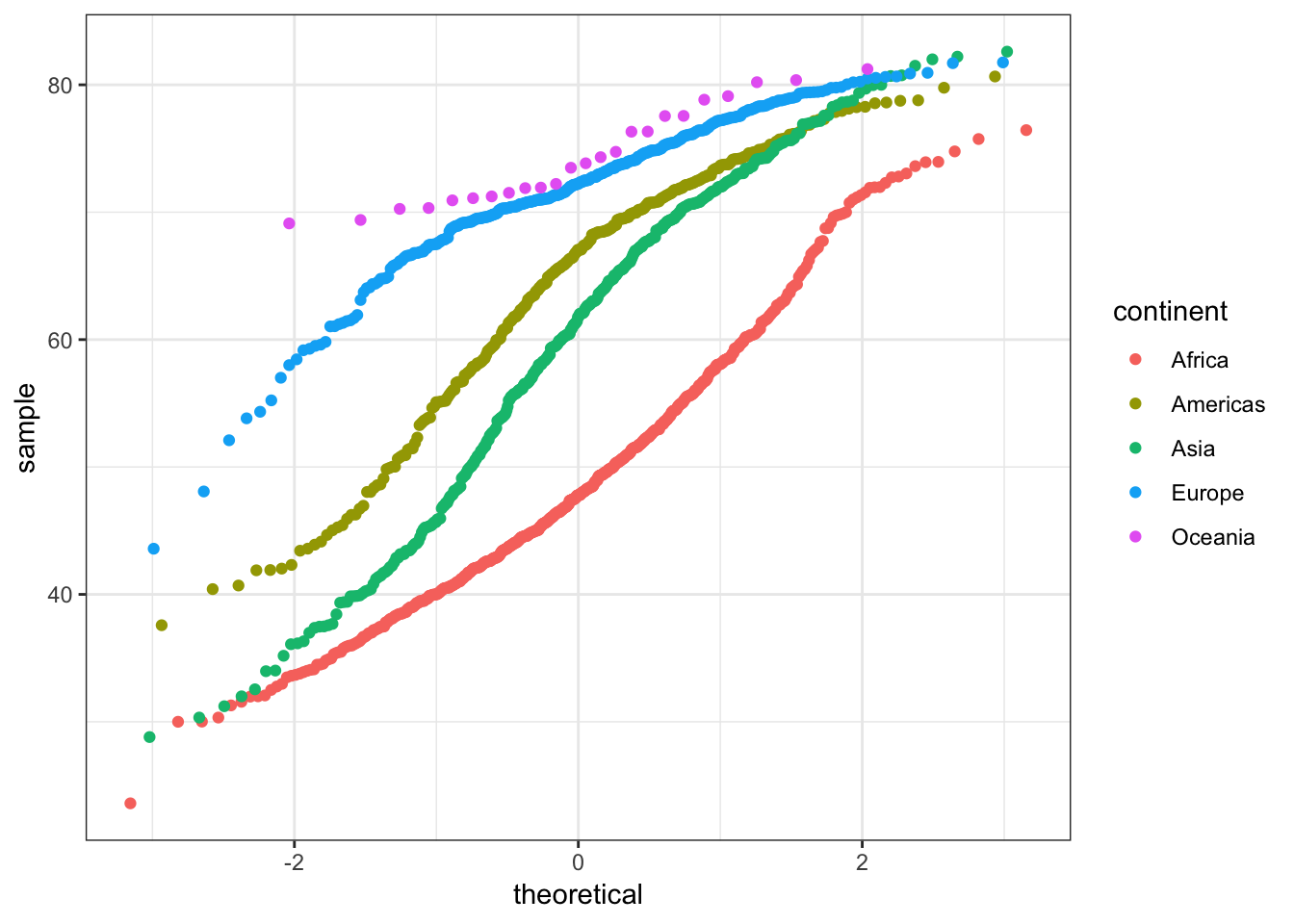# 14 Data Visualization Basics

## 14.1 Plots

• Single variables:
• Barplot
• Boxplot
• Histogram
• Density plot
• Two or more variables:
• Side-by-Side Boxplots
• Stacked Barplot
• Scatterplot

## 14.2 R Base Graphics

• We’ll first plodding through “R base graphics”, which means graphics functions that come with R.
• By default they are very simple. However, they can be customized a lot, but it takes a lot of work.
• Also, the syntax varies significantly among plot types and some think the syntax is not user-friendly.
• We will consider a very highly used graphics package next week, called ggplot2 that provides a “grammar of graphics”. It hits a sweet spot of “flexibility vs. complexity” for many data scientists.

For all of the plotting functions covered below, read the help files.

> ?barplot
> ?boxplot
> ?hist
> ?density
> ?plot
> ?legend

> cyl_tbl <- table(mtcars$cyl) > barplot(cyl_tbl, xlab="Cylinders", ylab="Count")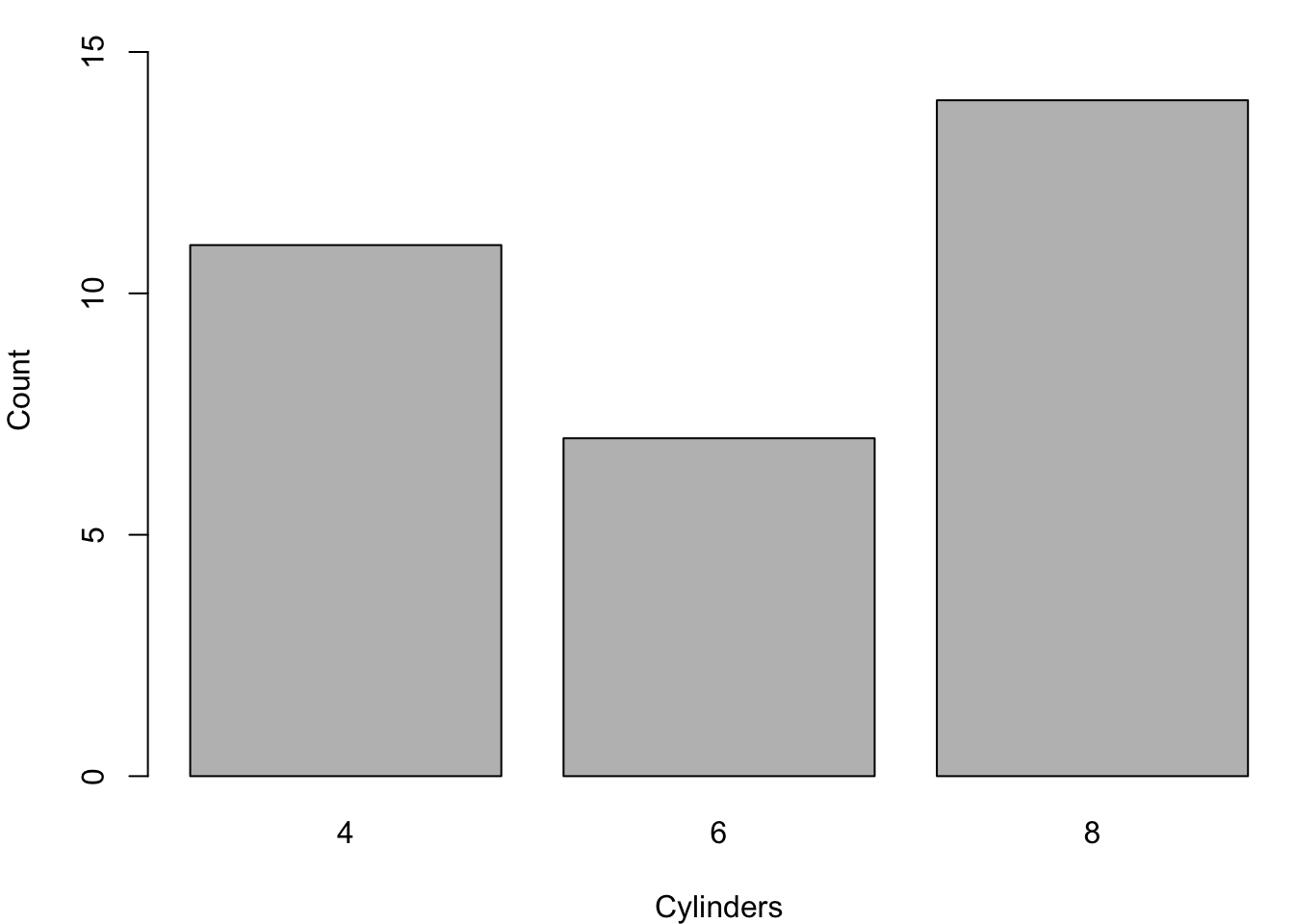## 14.5 Boxplot > boxplot(mtcars$mpg, ylab="MPG", col="lightgray")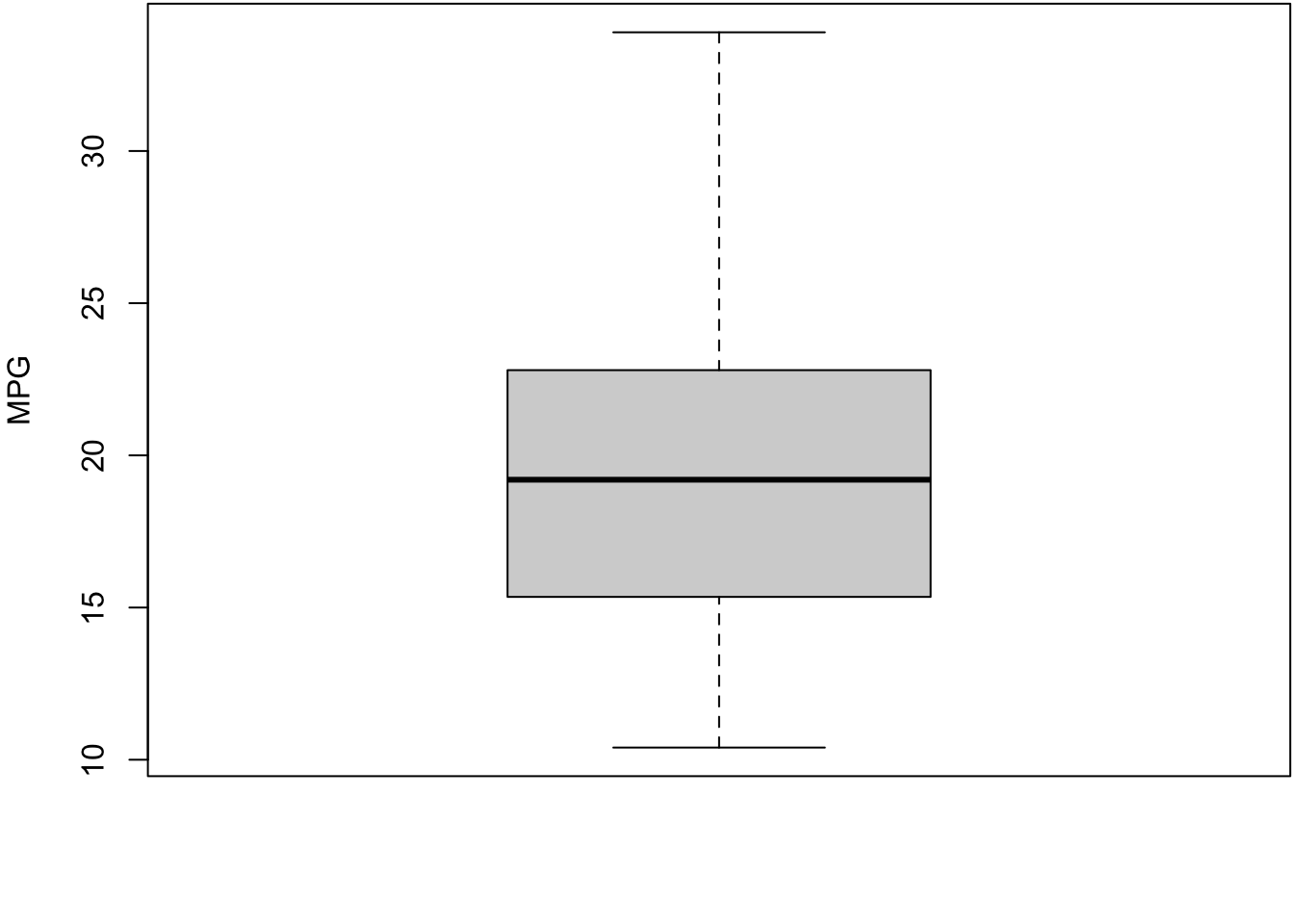## 14.6 Constructing Boxplots

• The top of the box is Q3
• The line through the middle of the box is the median
• The bottom of the box is Q1
• The top whisker is the minimum of Q3 + 1.5 $$\times$$ IQR or the largest data point
• The bottom whisker is the maximum of Q1 - 1.5 $$\times$$ IQR or the smallest data point
• Outliers lie outside of (Q1 - 1.5 $$\times$$ IQR) or (Q3 + 1.5 $$\times$$ IQR), and they are shown as points
• Outliers are calculated using the fivenum() function

> boxplot(mtcars$wt, ylab="Weight (1000 lbs)", + col="lightgray")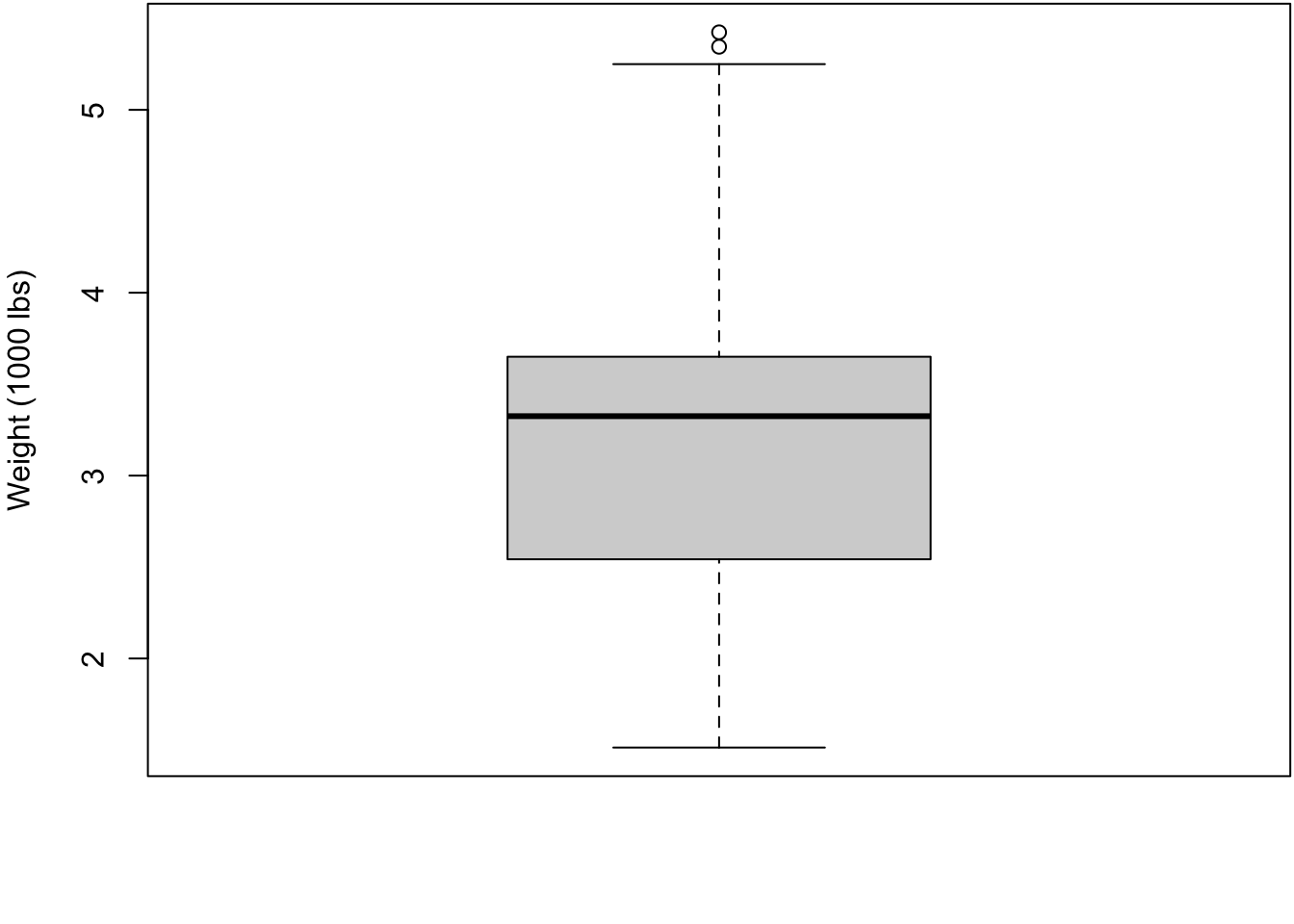## 14.8 Histogram > hist(mtcars$mpg, xlab="MPG", main="", col="lightgray")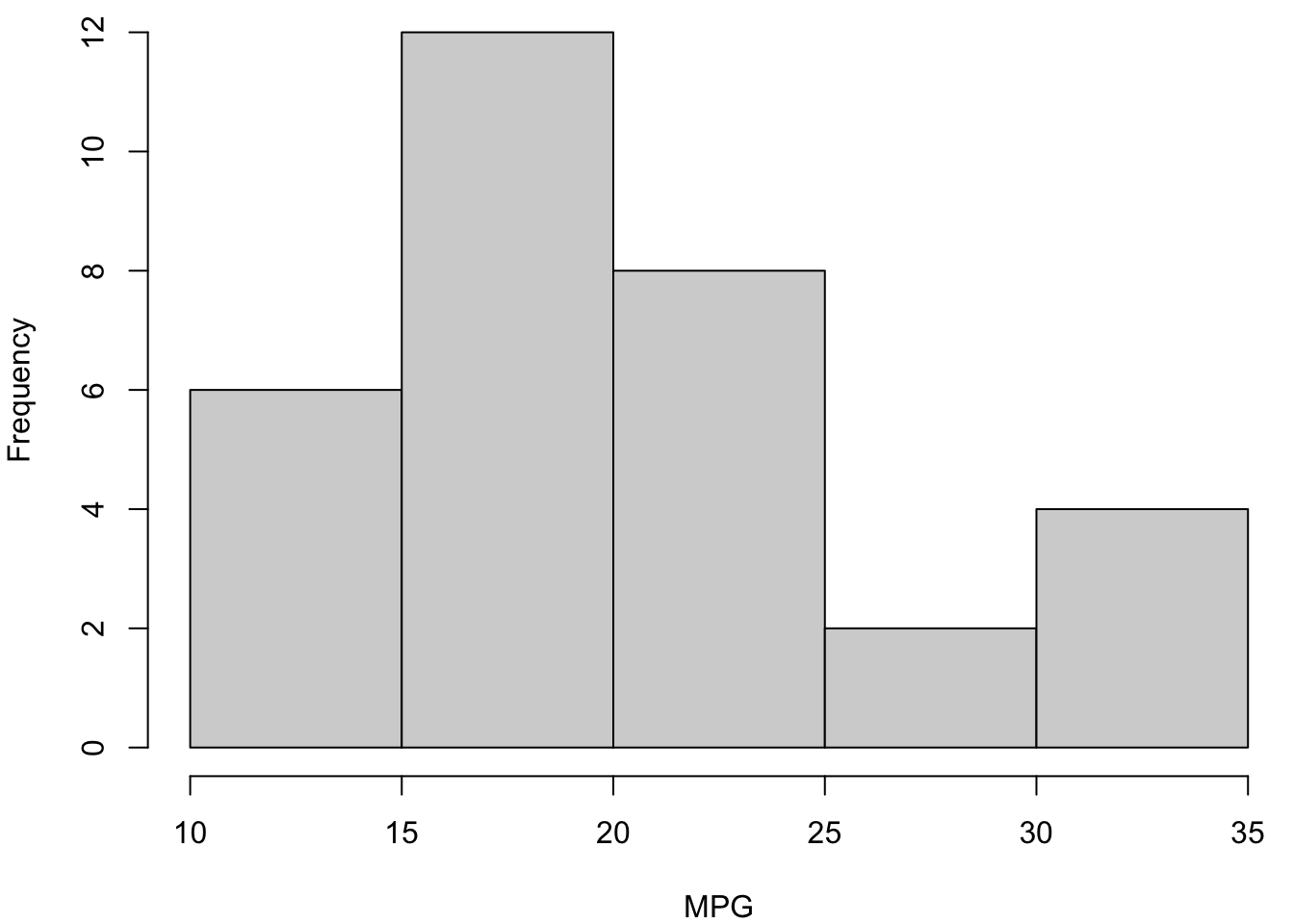> polygon(density(mtcars$mpg), col="lightgray", border="black")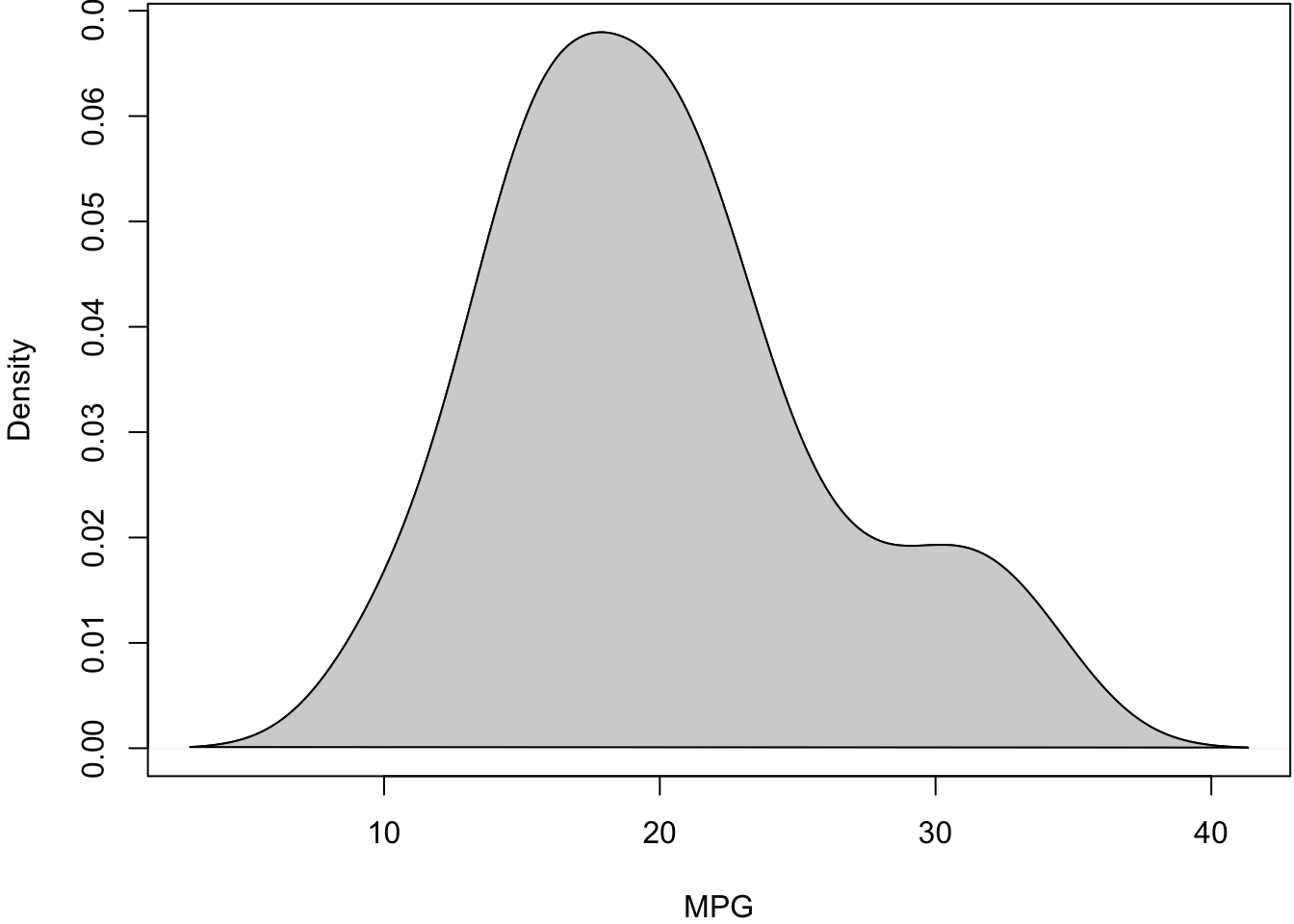## 14.11 Boxplot (Side-By-Side) > boxplot(mpg ~ cyl, data=mtcars, xlab="Cylinders", + ylab="MPG", col="lightgray")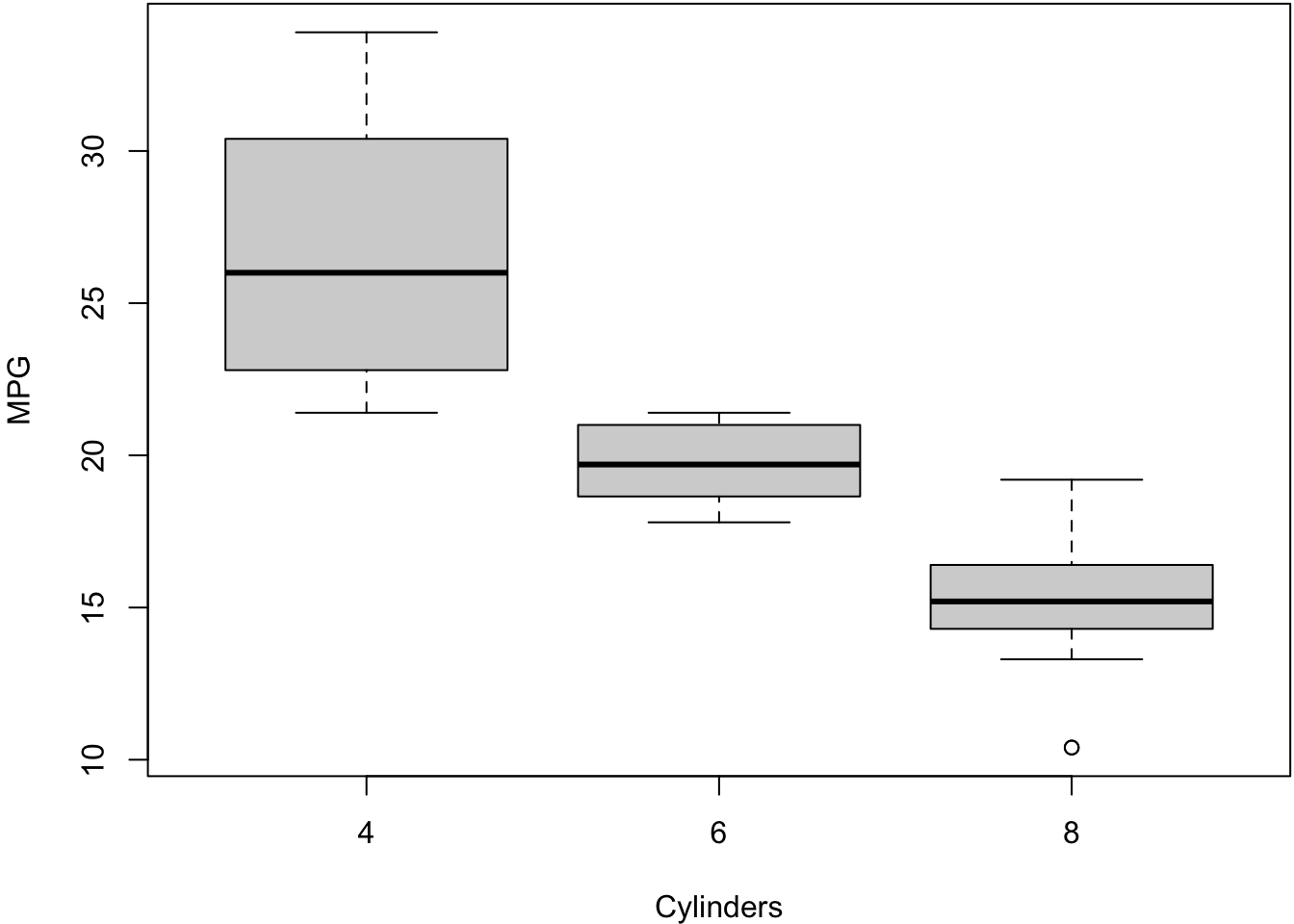## 14.12 Stacked Barplot > counts <- table(mtcars$cyl, mtcars$gear) > counts 3 4 5 4 1 8 2 6 2 4 1 8 12 0 2 > barplot(counts, main="Number of Gears and Cylinders", + xlab="Gears", col=c("blue","red", "lightgray")) > legend(x="topright", title="Cyl", + legend = rownames(counts), + fill = c("blue","red", "lightgray"))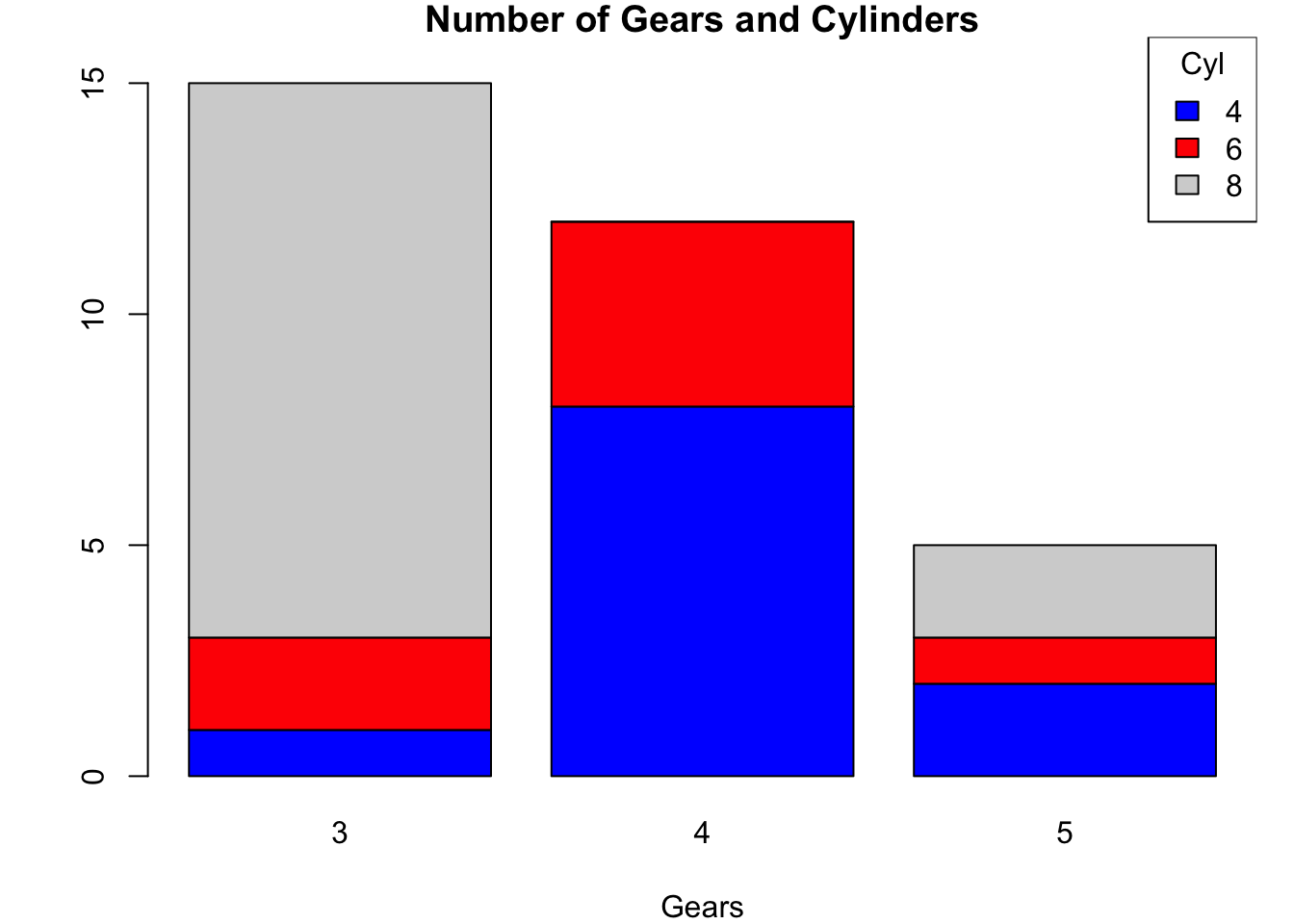## 14.13 Scatterplot > plot(mtcars$wt, mtcars$mpg, xlab="Weight (1000 lbs)", + ylab="MPG")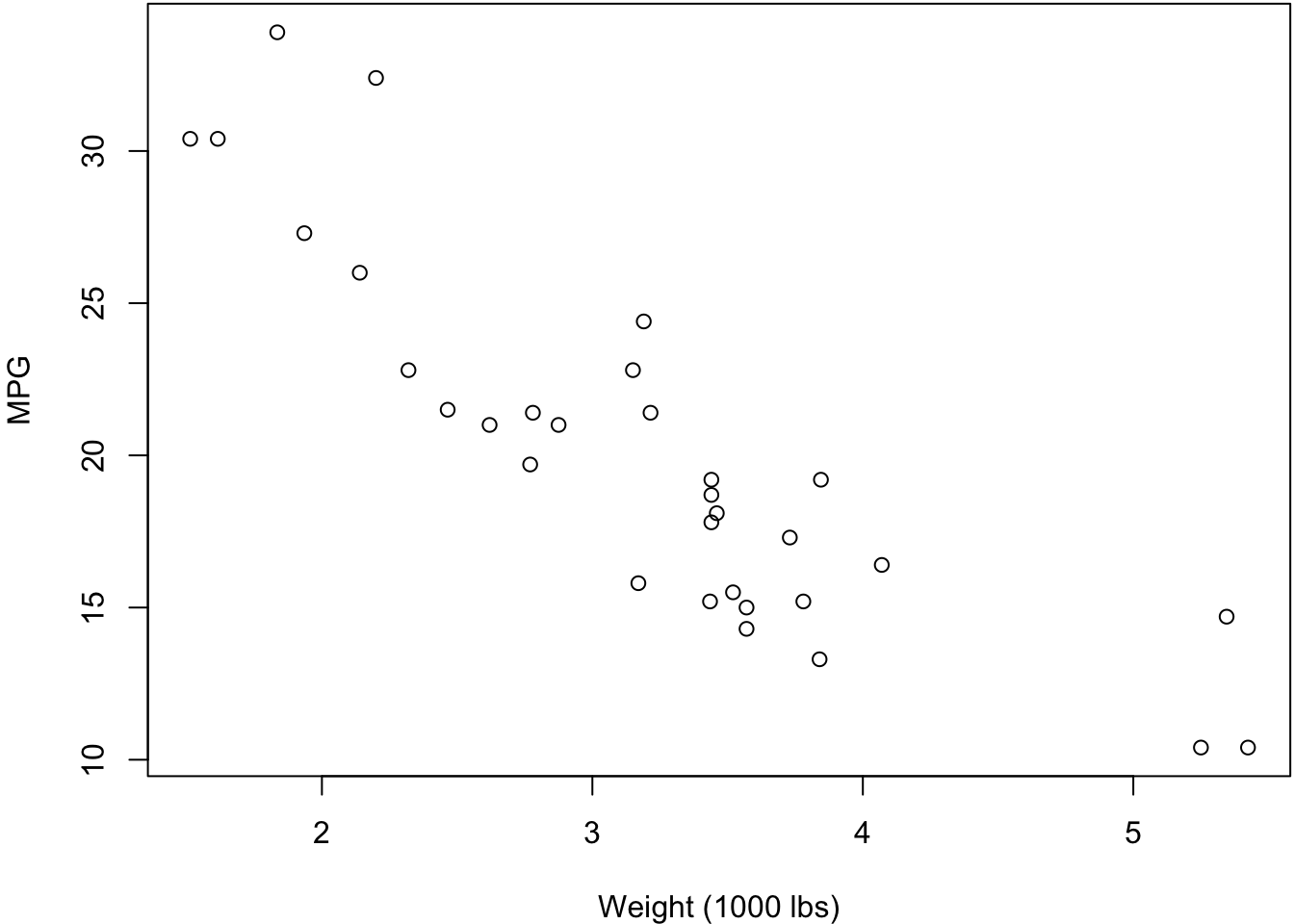## 14.14 Quantile-Quantile Plots Quantile-quantile plots display the quantiles of: 1. two samples of data 2. a sample of data vs a theoretical distribution The first type allows one to assess how similar the distributions are of two samples of data. The second allows one to assess how similar a sample of data is to a theoretical distribution (often Normal with mean 0 and standard deviation 1). > qqnorm(mtcars$mpg, main=" ")
> qqline(mtcars\$mpg) # line through Q1 and Q3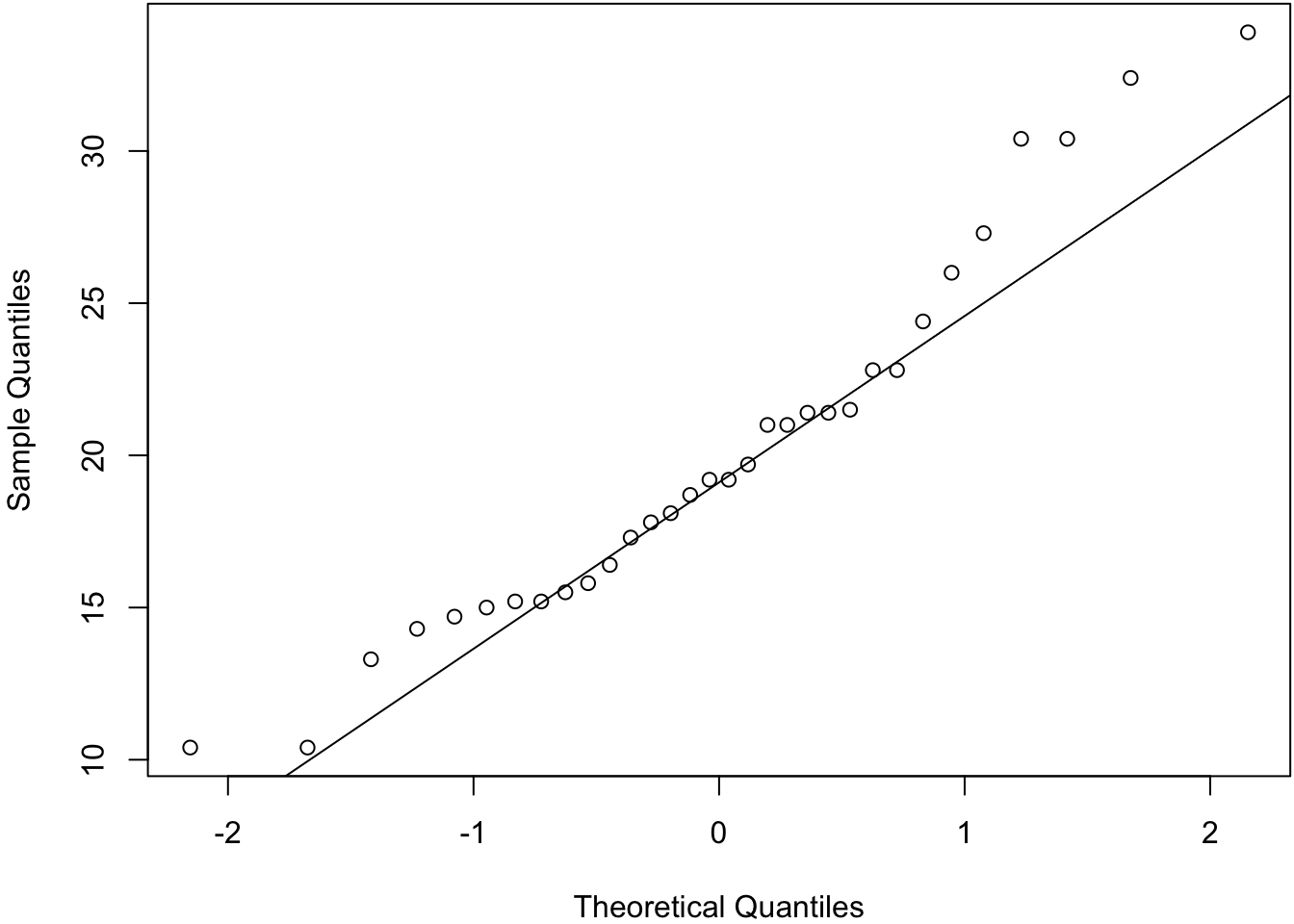> before1980 <- gapminder %>% filter(year < 1980) %>%
+   select(lifeExp) %>% unlist()
> after1980 <- gapminder %>% filter(year > 1980) %>%
+   select(lifeExp) %>% unlist()
> qqplot(before1980, after1980); abline(0,1)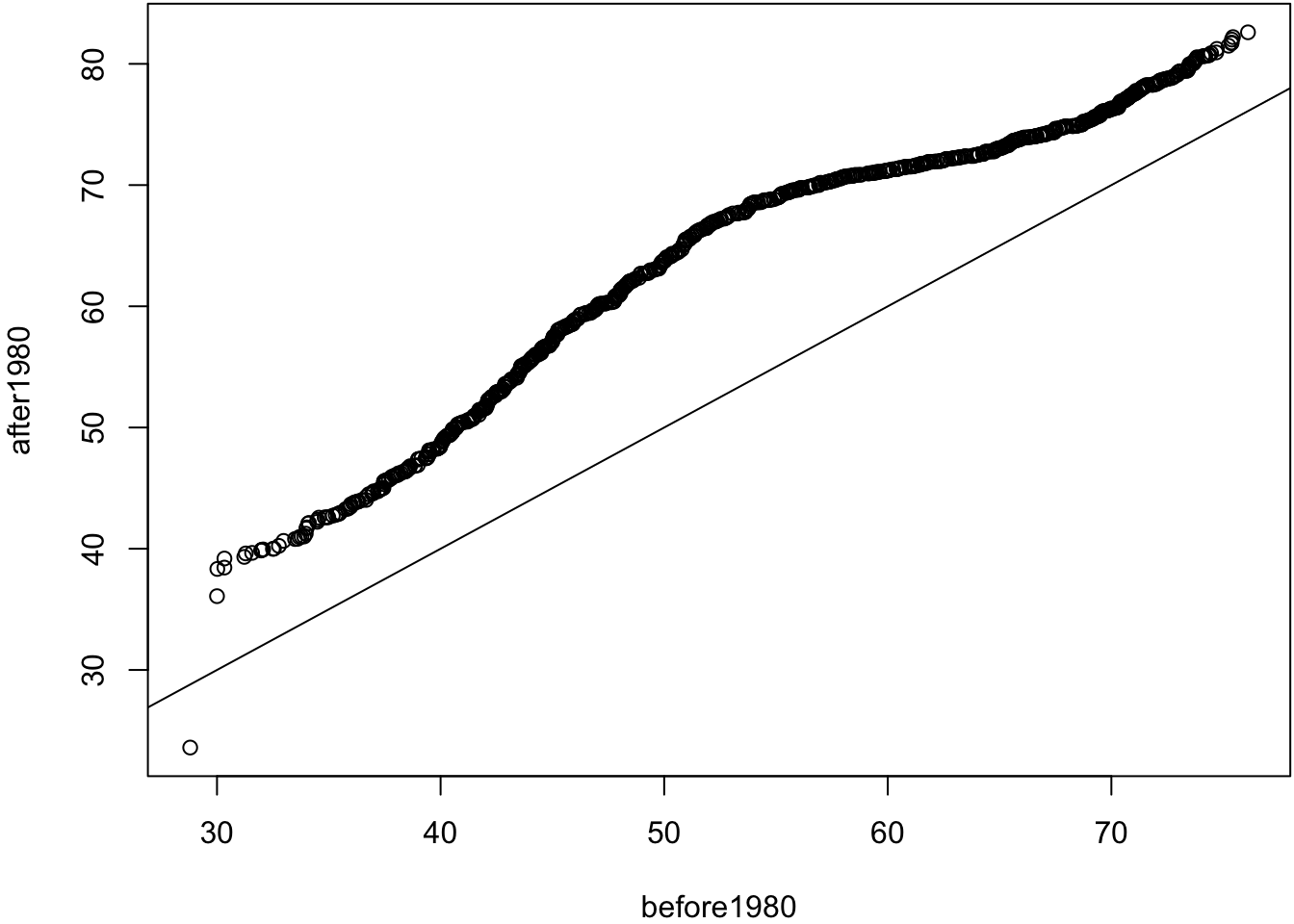> ggplot(mtcars) + stat_qq(aes(sample = mpg))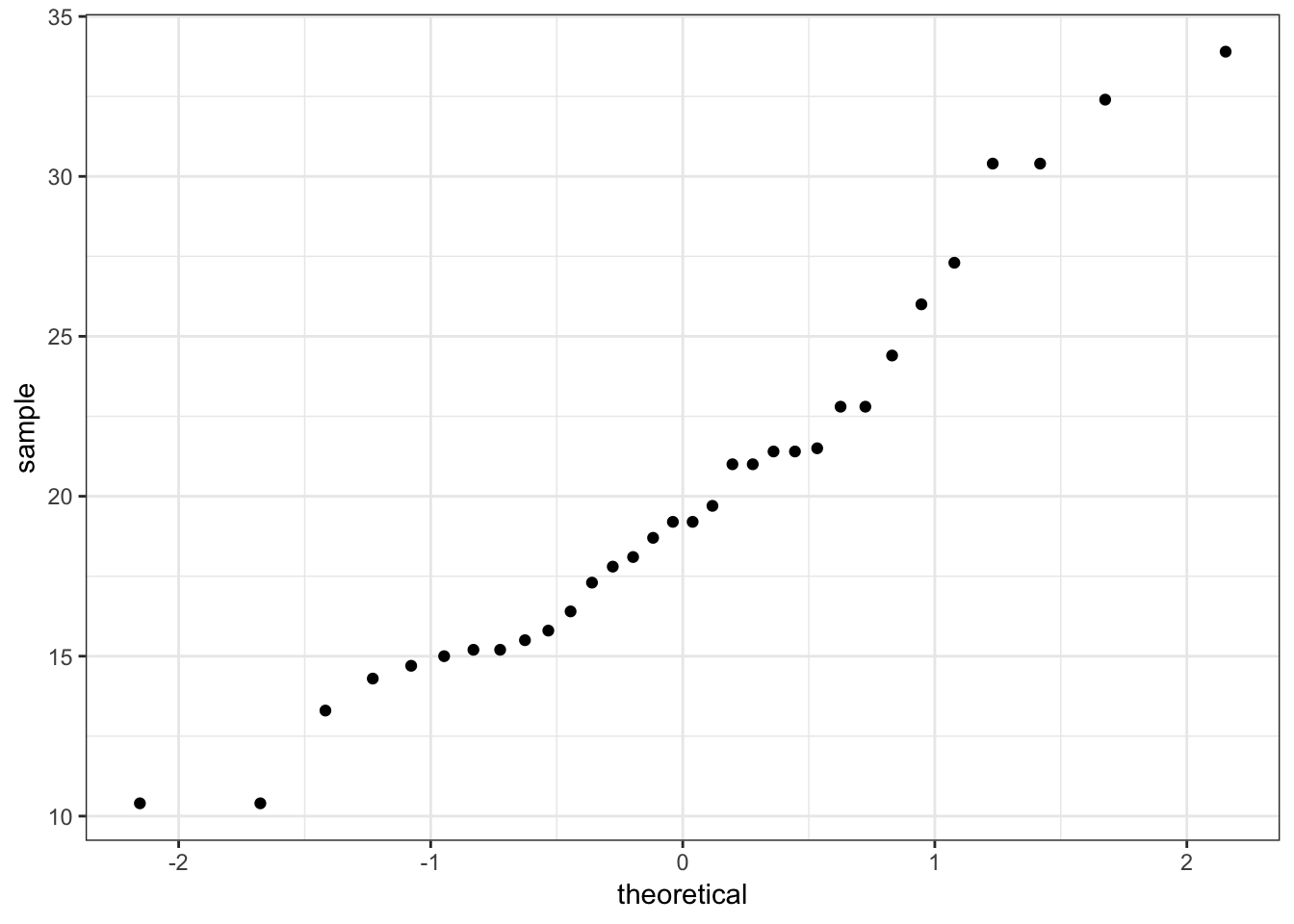> ggplot(gapminder) + stat_qq(aes(sample=lifeExp))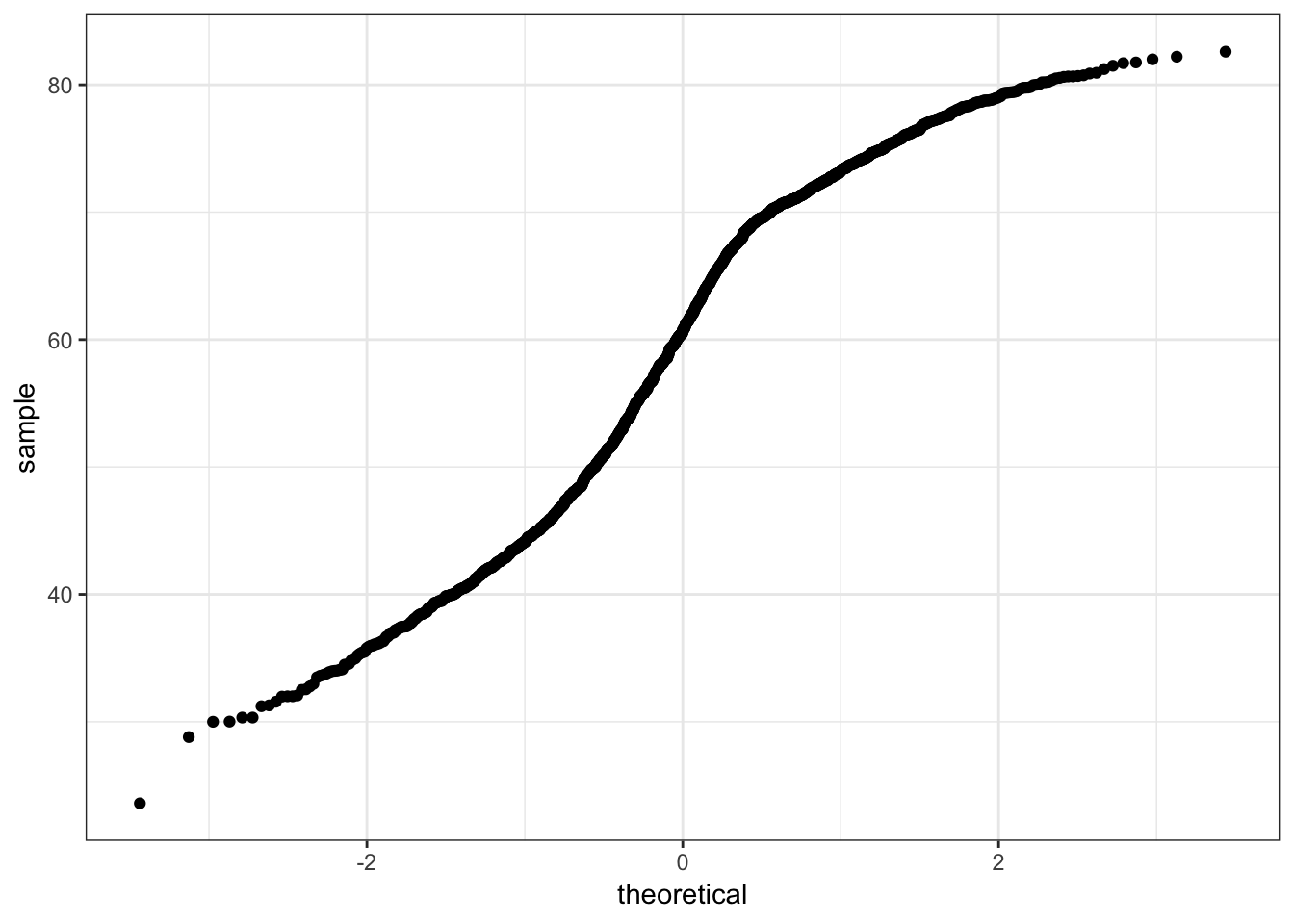> ggplot(gapminder) +
+   stat_qq(aes(sample=lifeExp, color=continent))